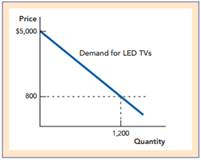### Create an Account

Home / Questions / Lets think about the demand for LED TVs a If the price for a 60inch LED TV is \$800 and Ne...

# Lets think about the demand for LED TVs a If the price for a 60inch LED TV is \$800 and Newhart would be willing to pay \$3000 what is Newharts consumer surplus b Consider the following

Let’s think about the demand for LED TVs.

a. If the price for a 60-inch LED TV is \$800, and Newhart would be willing to pay \$3,000, what is Newhart’s consumer surplus?

b. Consider the following figure for the total demand for LED TVs. At \$800 per TV, 1,200 TVs were demanded. What would be the total consumer surplus? Calculate the total, and identify it on the diagram.c. Where is Newhart in the figure?

Apr 21 2020 View more View LessSubscribe To Get Solution